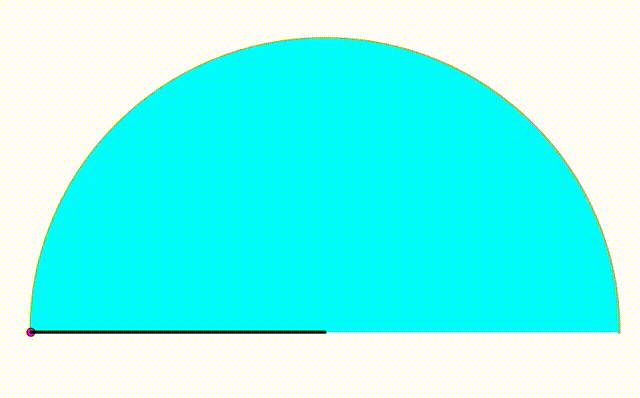# Dynamic Geometry: P80

Geometry Level pendingThe diagram shows an orange semicircle with radius $1$. The cyan and green semicircle are growing and shrinking freely on the black semicirle's diameter. The pink circle is internally tangent to the black semicircle and tangent to both green and cyan semicircle. Using their three centers, we draw a black triangle. When the ratio of its area to its perimeter is equal to $\dfrac{3}{28}$, the ratio of the larger semicircle's radius to the radius of the smaller semicircle can be expressed as $\dfrac{p}{q}$, where $p$ and $q$ are coprime positive integers. Find $p+q$.

×